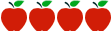### Grade 6: Rate, Ratio and Proportional Reasoning

Rating:#### Common Core Standards

Content Standards

6.RP.1 - Understand the concept of a ratio and use ratio language to describe a ratio relationship between two quantities.

6.RP.2 - Understand the concept of a unit rate a/b associated with a ratio a:b with b ≠ 0, and use rate language in the context of a ratio relationship.

6.RP.3 - Use ratio and rate reasoning to solve real-world and mathematical problems, e.g., by reasoning about tables of equivalent ratios, tape diagrams, double number line diagrams, or equations.

6.RP.3a - Make tables of equivalent ratios relating quantities with whole-number measurements, find missing values in the tables, and plot the pairs of values on the coordinate plane. Use tables to compare ratios.

6.RP.3b - Solve unit rate problems including those involving unit pricing and constant speed.

6.RP.3c - Find a percent of a quantity as a rate per 100; solve problems involving finding the whole, given a part and the percent.

6.RP.3d - Use ratio reasoning to convert measurement units; manipulate and transform units appropriately when multiplying or dividing quantities.

Standards of Mathematical Practice

MP.1  Make sense of problems and persevere in solving them.

MP.2  Reason abstractly and quantitatively.

MP.3  Construct viable arguments and critique the reasoning of others.

MP.4  Model with mathematics.

#### Description of Unit

This unit “Rate, Ratio and Proportional Reasoning Using Equivalent Fractions” from the Georgia DOE is a collection of 6 tasks encompassing the major concepts of ratios and rates using equivalent fractions. The overall unit is paced to 3 - 4 weeks. In this unit, students will gain a deeper understanding of proportional reasoning, understanding that a ratio is a comparison of two numbers or quantities. Students will develop and use multiplicative thinking and find percent using the same process for solving rates and proportions. Lastly, students will solve real-life problems involving measurement units that need to be converted.

#### Cautions

Connecticut teachers should be aware that the unit does not fully address the scope of 6.RP.3a, using ratio tables and tape diagrams to solve problems.  The unit does not include the following components:

• differentiation or supports for students working above/below grade level, English language learners, or students with disabilities.
• use of technology or media.
• explicit connections between the Standards for Mathematical Practice in the unit and the content.
• structures for implementing and completing performance tasks. There are no instructional notes (answer keys, rubrics, student work samples).

#### Rationale for Selection

This unit addresses one of the major areas of focus for this grade. The standards addressed in each task are identified at the beginning of the task; there is evidence of rigor and coherence within the Ratio and Proportional Reasoning Domain. The rigorous tasks are relevant and thought provoking; they encourage contextual understanding of the standards.